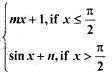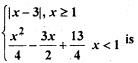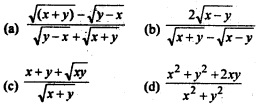# CBSEtips.in

## Thursday, 28 January 2021

### CBSE Class 12 Maths - MCQ and Online Tests - Unit 5 - Continuity and Differentiability

#### CBSE Class 12 Maths – MCQ and Online Tests – Unit 5 – Continuity and Differentiability

Every year CBSE conducts board exams for 12th standard. These exams are very competitive to all the students. So our website provides online tests for all the 12th subjects. These tests are also very effective and useful for those who preparing for competitive exams like NEET, JEE, CA etc. It can boost their preparation level and confidence level by attempting these chapter wise online tests.

These online tests are based on latest CBSE Class 12 syllabus. While attempting these our students can identify the weak lessons and continuously practice those lessons for attaining high marks. It also helps to revise the NCERT textbooks thoroughly.

#### CBSE Class 12 Maths – MCQ and Online Tests – Unit 5 – Continuity and Differentiability

Question 1.
If f(x) =is continuous at x = $$\frac{π}{2}$$, then
(a) m = 1, n = 0
(b) m = $$\frac{nπ}{2}$$ + 1
(c) n = $$\frac{mπ}{2}$$
(d) m = n = $$\frac{π}{2}$$

Answer: (c) n = $$\frac{mπ}{2}$$

Question 2.
Let f(x) = |sin x| Then
(a) f is everywhere differentiable
(b) f is everywhere continuous but not differentiable at x = nπ, n ∈ Z
(c) f is everywhere continuous but no differentiable at x = (2n + 1) $$\frac{π}{2}$$ n ∈ Z
(d) None of these

Answer: (b) f is everywhere continuous but not differentiable at x = nπ, n ∈ Z

Question 3.
If y = $$\sqrt{sin x+y}$$ then $$\frac{dy}{dx}$$ is equal to
(a) $$\frac{cosx}{2y-1}$$
(b) $$\frac{cosx}{1-2y}$$
(c) $$\frac{sinx}{1-xy}$$
(d) $$\frac{sinx}{2y-1}$$

Answer: (a) $$\frac{cosx}{2y-1}$$

Question 4.
If sec ($$\frac{x-y}{x+y}$$) = a then $$\frac{dy}{dx}$$ is
(a) –$$\frac{y}{x}$$
(b) $$\frac{x}{y}$$
(c) –$$\frac{x}{y}$$
(d) $$\frac{y}{x}$$

Answer: (d) $$\frac{y}{x}$$

Question 5.
The derivative of cos-1 (2x² – 1) w.r.t cos-1 x is
(a) 2
(b) $$\frac{-1}{2\sqrt{1-x^2}}$$
(c) $$\frac{2}{x}$$
(d) 1 – x²

Question 6.
If x = t², y = t³, then $$\frac{d^2y}{dx^2}$$
(a) $$\frac{3}{2}$$
(b) $$\frac{3}{4t}$$
(c) $$\frac{3}{2t}$$
(d) $$\frac{3}{4t}$$

Answer: (b) $$\frac{3}{4t}$$

Question 7.
If y = log($$\frac{1-x^2}{1+x^2}$$), then $$\frac{dy}{dx}$$ is equal to
(a) $$\frac{4x^3}{1-x^4}$$
(b) $$\frac{-4x}{1-x^4}$$
(c) $$\frac{1}{4-x^4}$$
(d) $$\frac{-4x^3}{1-x^4}$$

Answer: (b) $$\frac{-4x}{1-x^4}$$

Question 8.
The value of c in Rolle’s theorem for the function f(x) = x³ – 3x in the interval [o, √3] is
(a) 1
(b) -1
(c) $$\frac{3}{2}$$
(d) $$\frac{1}{3}$$

Question 9.
If f (x) = 2x and g (x) = $$\frac{x^2}{2}$$ + 1, then’which of the following can be a discontinuous function
(a) f(x) + g(x)
(b) f(x) – g(x)
(c) f(x).g(x)
(d) $$\frac{g(x)}{f(x)}$$

Answer: (d) $$\frac{g(x)}{f(x)}$$

Question 10.
For the function f(x) = x + $$\frac{1}{x}$$, x ∈ [1, 3] the value of c for mean value theorem is
(a) 1
(b) √3
(c) 2
(d) None of these

Question 11.
Let function f (x) =(a) continuous at x = 1
(b) differentiable at x = 1
(c) continuous at x = -3
(d) All of these

Question 12.
If x sin (a + y) = sin y, then $$\frac{dy}{dx}$$ is equal to
(a) $$\frac{sin^2(a+y)}{sin a}$$
(b) $$\frac{sin a}{sin^2(a+y)}$$
(c) $$\frac{sin(a+y)}{sin a}$$
(d) $$\frac{sin a}{sin(a+y)}$$

Answer: (a) $$\frac{sin^2(a+y)}{sin a}$$

Question 13.
The set of points where the function f given by f (x) =| 2x – 1| sin x is differentiable is
(a) R
(b) R = {$$\frac{1}{2}$$}
(c) (0, ∞)
(d) None of these

Answer: (b) R = {$$\frac{1}{2}$$}

Question 14.
If sec($$\frac{x^2-2x}{x^2+1}$$) – y then $$\frac{dy}{dx}$$ is equal to
(a) $$\frac{y*2}{x^2}$$
(b) $$\frac{2y\sqrt{y^2-1}(x^2+x-1)}{(x^2+1)^2}$$
(c) $$\frac{(x^2+x-1)}{y\sqrt{y^2-1}}$$
(d) $$\frac{x^2-y^2}{x^2+y^2}$$

Answer: (b) $$\frac{2y\sqrt{y^2-1}(x^2+x-1)}{(x^2+1)^2}$$

Question 15.
If y = tan-1($$\frac{sinx+cosx}{cox-sinx}$$) then $$\frac{dy}{dx}$$ is equal to
(a) $$\frac{1}{2}$$
(b) $$\frac{π}{4}$$
(c) 0
(d) 1

Question 16.
The function f(x) = cot x is discontinuous on the set
(a) {x = nπ, n ∈ Z}
(b) {x = 2nπ, n ∈ Z}
(c) {x = (2n + 1) $$\frac{π}{2}$$ n ∈ Z}
(d) {x – $$\frac{nπ}{2}$$ n ∈ Z}

Answer: (a) {x = nπ, n ∈ Z}

Question 17.
Differential coefficient of $$\sqrt{sec√x}$$ is
(a) $$\frac{1}{4√x}$$ = sec √x sin √x
(b) $$\frac{1}{4√x}$$ = (sec√x)3/2 sin√x
(c) $$\frac{1}{2}$$ √x sec√x sin √x.
(d) $$\frac{1}{2}$$√x (sec√x)3/2 sin√x

Answer: (b) $$\frac{1}{4√x}$$ = (sec√x)3/2 sin√x

Question 18.
If f(x) = $$\frac{\sqrt{4+x}-2}{x}$$ x ≠ 0 be continuous at x = 0, then f(o) =
(a) $$\frac{1}{2}$$
(b) $$\frac{1}{4}$$
(c) 2
(d) $$\frac{3}{2}$$

Answer: (b) $$\frac{1}{4}$$

Question 19.
let f(2) = 4 then f”(2) = 4 then $$_{x→2}^{lim}$$ $$\frac{xf(2)-2f(x)}{x-2}$$ is given by
(a) 2
(b) -2
(c) -4
(d) 3

Question 20.
The function f(x) = $$\frac{4-x^2}{4x-x^3}$$ is
(a) discontinuous at only one point at x = 0
(b) discontinuous at exactly two points
(c) discontinuous at exactly three points
(d) None of these

Answer: (a) discontinuous at only one point at x = 0

Question 21.
It is given that f'(a) exists, then $$_{x→2}^{lim}$$ [/latex] $$\frac{xf(a)-af(x)}{(x-a)}$$ is equal to
(a) f(a) – af'(a)
(b) f'(a)
(c) -f’(a)
(d) f (a) + af'(a)

Question 22.
If y = ax² + b, then $$\frac{dy}{dx}$$ at x = 2 is equal to ax
(a) 4a
(b) 3a
(c) 2a
(d) None of these

Question 23.
If x $$\sqrt{1+y}+y\sqrt{1+x}$$ = 0, then $$\frac{dy}{dx}$$ =
(a) $$\frac{x+1}{x}$$
(b) $$\frac{1}{1+x}$$
(c) $$\frac{-1}{(1+x)^2}$$
(d) $$\frac{x}{1+x}$$

Answer: (c) $$\frac{-1}{(1+x)^2}$$

Question 24.
Let f be defined on [-5, 5] as
f(x) = {$$_{-x, if x is irrational}^{x, if x is rational}$$ Then f(x) is
(a) continuous at every x except x = 0
(b) discontinuous at everyx except x = 0
(c) continuous everywhere
(d) discontinuous everywhere

Answer: (b) discontinuous at everyx except x = 0

Question 25.
If y = x tan y, then $$\frac{dy}{dx}$$ =
(a) $$\frac{tan x}{x-x^2-y^2}$$
(b) $$\frac{y}{x-x^2-y^2}$$
(c) $$\frac{tan y}{y-x}$$
(d) $$\frac{tan x}{x-y^2}$$

Answer: (b) $$\frac{y}{x-x^2-y^2}$$

Question 26.
If f(x) = $$\sqrt{25-x^2}$$, then $$_{x→2}^{lim}$$$$\frac{f(x)-f(1)}{x-1}$$ is equal to
(a) $$\frac{1}{24}$$
(b) $$\frac{1}{5}$$
(c) –$$\sqrt{24}$$
(d) $$\frac{1}{\sqrt{24}}$$

Answer: (d) $$\frac{1}{\sqrt{24}}$$

Question 27.
If y = (1 + x) (1 + x²) (1 + x4) …….. (1 + x2n), then the value of $$\frac{dy}{dx}$$ at x = 0 is
(a) 0
(b) -1
(c) 1
(d) None of these

Question 28.
If f(x) = $$\frac{5x}{(1-x)^{2/3}}$$ + cos² (2x + 1), then f'(0) =
(a) 5 + 2 sin 2
(b) 5 + 2 cos 2
(c) 5 – 2 sin 2
(d) 5 – 2 cos 2

Answer: (c) 5 – 2 sin 2

Question 29.
If f(x) = $$\sqrt{1+cos^2(x^2)}$$, then the value of f’ ($$\frac{√π}{2}$$) is
(a) $$\frac{√π}{6}$$
(b) –$$\frac{√π}{6}$$
(c) $$\frac{1}{√6}$$
(d) $$\frac{π}{√6}$$

Answer: (b) –$$\frac{√π}{6}$$

Question 30.
If xmyn = (x + y)m+n, then $$\frac{dy}{dx}$$ is equal to
(a) $$\frac{x+y}{xy}$$
(b) xy
(c) $$\frac{x}{y}$$
(d) $$\frac{y}{x}$$

Answer: (d) $$\frac{y}{x}$$

Question 31.
If $$\sqrt{(x+y)}$$ + $$\sqrt{(y-x)}$$ = a, then $$\frac{dy}{dx}$$Answer: (a) $$\frac{\sqrt{(x+y)}-\sqrt{(y-x)}}{\sqrt{y-x}+\sqrt{x+y}}$$

Question 32.
The function f(x) = e|x| is
(a) continuous everywhere but not differentiable at x = 0
(b) continuous and differentiable everywhere
(c) not continuous at x = 0
(d) None of these

Answer: (a) continuous everywhere but not differentiable at x = 0

Question 33.
If f(x) = x² sin$$\frac{1}{x}$$, where x ≠ 0, then the value of the function f(x) at x = 0, so that the function is continuous at x = 0 is
(a) 0
(b) -1
(c) 1
(d) None of these

Question 34.
Let f(x)={$$_{1-cos x, for x ≤ 0}^{sin x, for x > 0}$$ and g (x) = ex. Then the value of (g o f)’ (0) is
(a) 1
(b) -1
(c) 0
(d) None of these

Question 35.
If ax² + 2hxy + by² = 1, then $$\frac{dy}{dx}$$equals
(a) $$\frac{hx+by}{ax+by}$$
(b) $$\frac{ax+by}{hx+by}$$
(c) $$\frac{ax+hy}{hx+hy}$$
(d) $$\frac{-(ax+hy)}{hx+by}$$

Answer: (d) $$\frac{-(ax+hy)}{hx+by}$$

Share: